# Basic concept and first law of thermodynamics

2. Jul 2018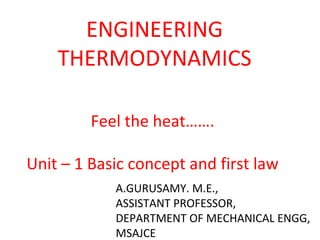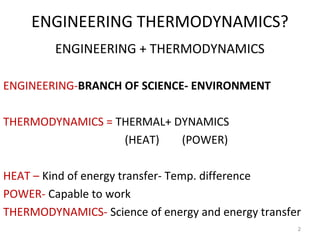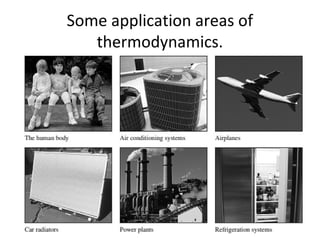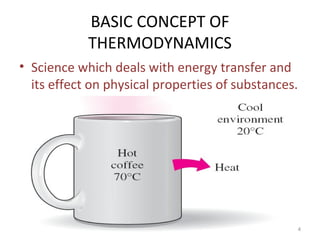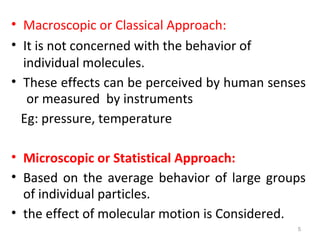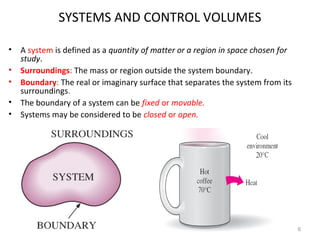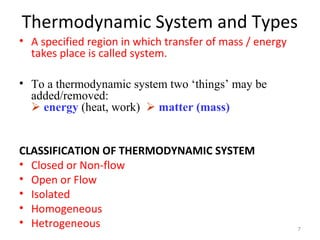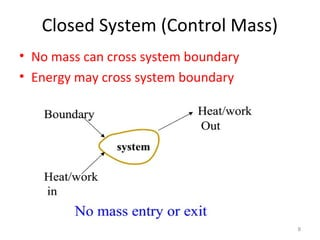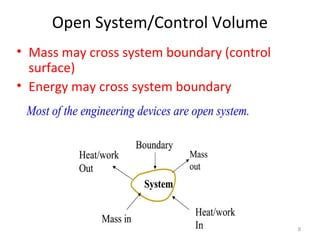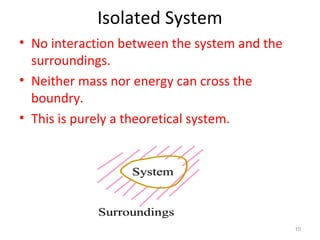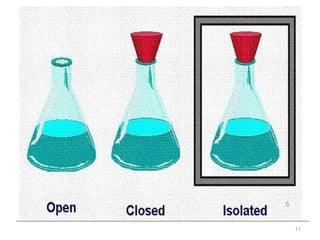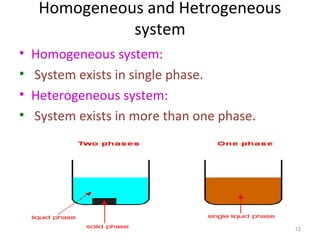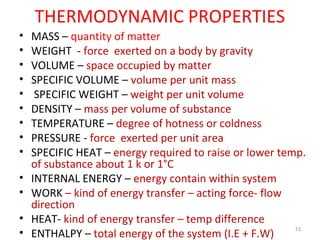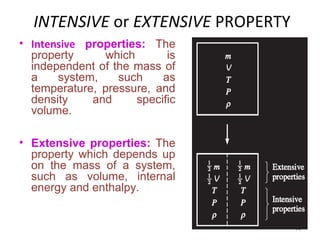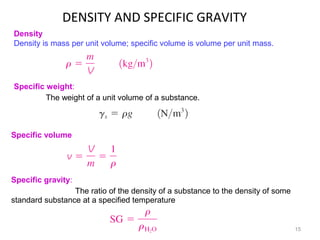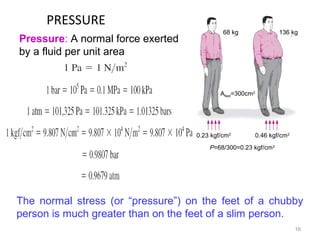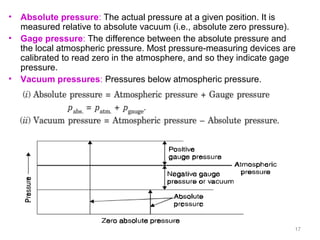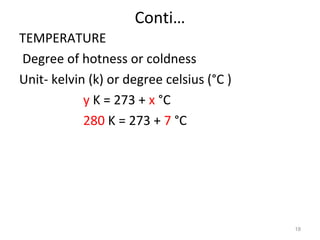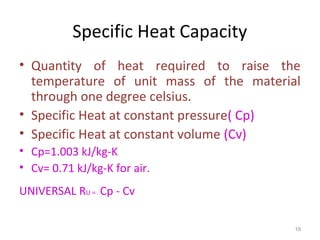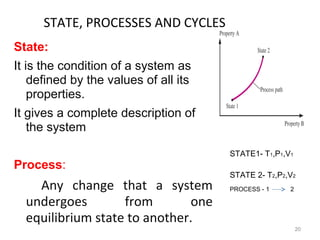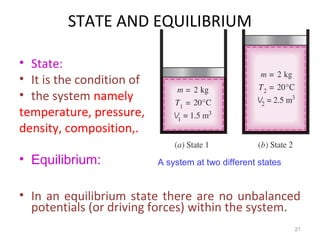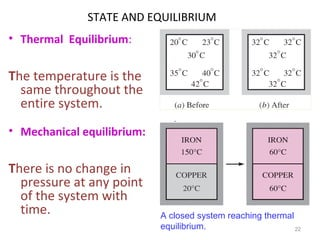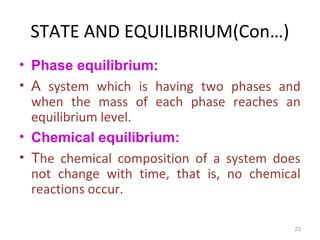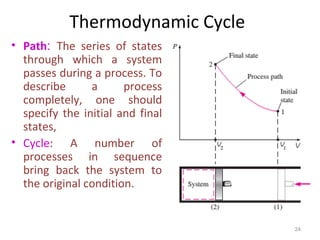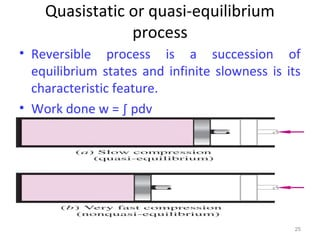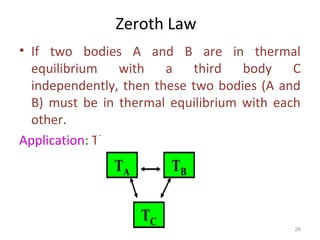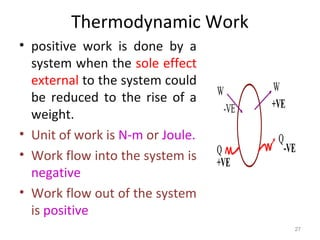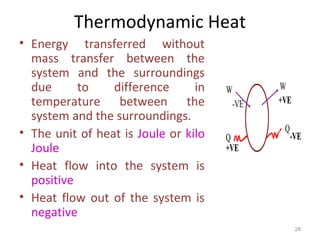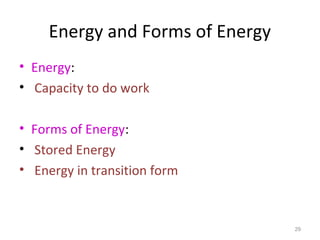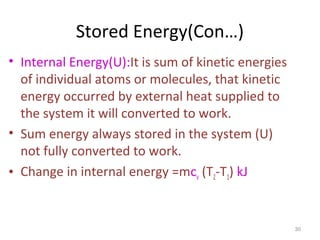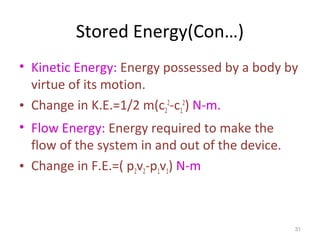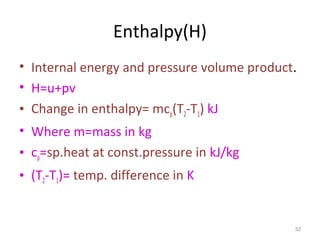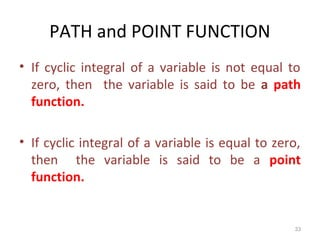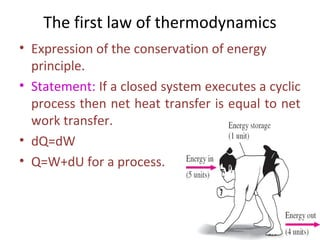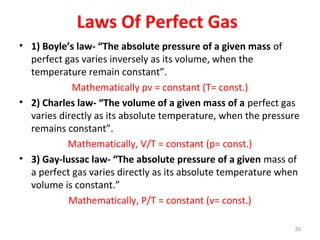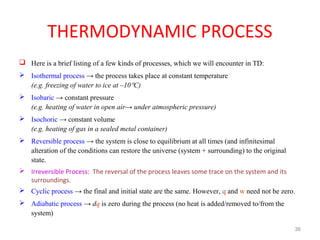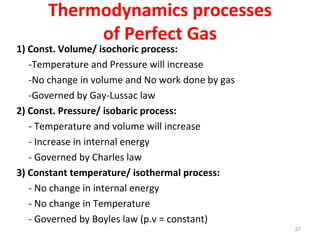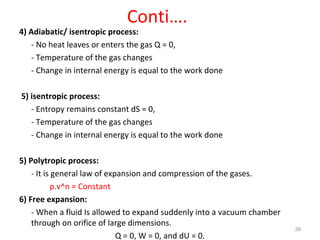1 von 39

### Basic concept and first law of thermodynamics

• 1. ENGINEERING THERMODYNAMICS Feel the heat……. Unit – 1 Basic concept and first law A.GURUSAMY. M.E., ASSISTANT PROFESSOR, DEPARTMENT OF MECHANICAL ENGG, MSAJCE
• 2. ENGINEERING THERMODYNAMICS? ENGINEERING + THERMODYNAMICS ENGINEERING-BRANCH OF SCIENCE- ENVIRONMENT THERMODYNAMICS = THERMAL+ DYNAMICS (HEAT) (POWER) HEAT – Kind of energy transfer- Temp. difference POWER- Capable to work THERMODYNAMICS- Science of energy and energy transfer 2
• 3. Some application areas of thermodynamics. 3
• 4. BASIC CONCEPT OF THERMODYNAMICS • Science which deals with energy transfer and its effect on physical properties of substances. 4
• 5. • Macroscopic or Classical Approach: • It is not concerned with the behavior of individual molecules. • These effects can be perceived by human senses or measured by instruments Eg: pressure, temperature • Microscopic or Statistical Approach: • Based on the average behavior of large groups of individual particles. • the effect of molecular motion is Considered. 5
• 6. SYSTEMS AND CONTROL VOLUMES • A system is defined as a quantity of matter or a region in space chosen for study. • Surroundings: The mass or region outside the system boundary. • Boundary: The real or imaginary surface that separates the system from its surroundings. • The boundary of a system can be fixed or movable. • Systems may be considered to be closed or open. 6
• 7. Thermodynamic System and Types • A specified region in which transfer of mass / energy takes place is called system. • To a thermodynamic system two ‘things’ may be added/removed:  energy (heat, work)  matter (mass) CLASSIFICATION OF THERMODYNAMIC SYSTEM • Closed or Non-flow • Open or Flow • Isolated • Homogeneous • Hetrogeneous 7
• 8. Closed System (Control Mass) • No mass can cross system boundary • Energy may cross system boundary 8
• 9. Open System/Control Volume • Mass may cross system boundary (control surface) • Energy may cross system boundary 9
• 10. Isolated System • No interaction between the system and the surroundings. • Neither mass nor energy can cross the boundry. • This is purely a theoretical system. 10
• 11. 11
• 12. Homogeneous and Hetrogeneous system • Homogeneous system: • System exists in single phase. • Heterogeneous system: • System exists in more than one phase. 12
• 13. THERMODYNAMIC PROPERTIES • MASS – quantity of matter • WEIGHT - force exerted on a body by gravity • VOLUME – space occupied by matter • SPECIFIC VOLUME – volume per unit mass • SPECIFIC WEIGHT – weight per unit volume • DENSITY – mass per volume of substance • TEMPERATURE – degree of hotness or coldness • PRESSURE - force exerted per unit area • SPECIFIC HEAT – energy required to raise or lower temp. of substance about 1 k or 1°C • INTERNAL ENERGY – energy contain within system • WORK – kind of energy transfer – acting force- flow direction • HEAT- kind of energy transfer – temp difference • ENTHALPY – total energy of the system (I.E + F.W) 13
• 14. INTENSIVE or EXTENSIVE PROPERTY • Intensive properties: The property which is independent of the mass of a system, such as temperature, pressure, and density and specific volume. • Extensive properties: The property which depends up on the mass of a system, such as volume, internal energy and enthalpy. 14
• 15. DENSITY AND SPECIFIC GRAVITY 15 Specific gravity: The ratio of the density of a substance to the density of some standard substance at a specified temperature Density Density is mass per unit volume; specific volume is volume per unit mass. Specific weight: The weight of a unit volume of a substance. Specific volume
• 16. PRESSURE 16 The normal stress (or “pressure”) on the feet of a chubby person is much greater than on the feet of a slim person. Pressure: A normal force exerted by a fluid per unit area 68 kg 136 kg Afeet=300cm2 0.23 kgf/cm2 0.46 kgf/cm2 P=68/300=0.23 kgf/cm2
• 17. • Absolute pressure: The actual pressure at a given position. It is measured relative to absolute vacuum (i.e., absolute zero pressure). • Gage pressure: The difference between the absolute pressure and the local atmospheric pressure. Most pressure-measuring devices are calibrated to read zero in the atmosphere, and so they indicate gage pressure. • Vacuum pressures: Pressures below atmospheric pressure. 17
• 18. Conti… TEMPERATURE Degree of hotness or coldness Unit- kelvin (k) or degree celsius (°C ) y K = 273 + x °C 280 K = 273 + 7 °C 18
• 19. Specific Heat Capacity • Quantity of heat required to raise the temperature of unit mass of the material through one degree celsius. • Specific Heat at constant pressure( Cp) • Specific Heat at constant volume (Cv) • Cp=1.003 kJ/kg-K • Cv= 0.71 kJ/kg-K for air. UNIVERSAL RU = Cp - Cv 19
• 20. STATE, PROCESSES AND CYCLES State: It is the condition of a system as defined by the values of all its properties. It gives a complete description of the system Process: Any change that a system undergoes from one equilibrium state to another. 20 STATE1- T1,P1,V1 STATE 2- T2,P2,V2 PROCESS - 1 2
• 21. STATE AND EQUILIBRIUM • State: • It is the condition of • the system namely temperature, pressure, density, composition,. • Equilibrium: • In an equilibrium state there are no unbalanced potentials (or driving forces) within the system. 21 A system at two different states
• 22. STATE AND EQUILIBRIUM • Thermal Equilibrium: The temperature is the same throughout the entire system. • Mechanical equilibrium: There is no change in pressure at any point of the system with time. 22 A closed system reaching thermal equilibrium. .
• 23. STATE AND EQUILIBRIUM(Con…) • Phase equilibrium: • A system which is having two phases and when the mass of each phase reaches an equilibrium level. • Chemical equilibrium: • The chemical composition of a system does not change with time, that is, no chemical reactions occur. 23
• 24. Thermodynamic Cycle • Path: The series of states through which a system passes during a process. To describe a process completely, one should specify the initial and final states, • Cycle: A number of processes in sequence bring back the system to the original condition. 24
• 25. Quasistatic or quasi-equilibrium process • Reversible process is a succession of equilibrium states and infinite slowness is its characteristic feature. • Work done w = ∫ pdv 25
• 26. Zeroth Law • If two bodies A and B are in thermal equilibrium with a third body C independently, then these two bodies (A and B) must be in thermal equilibrium with each other. Application: Thermometer 26
• 27. Thermodynamic Work • positive work is done by a system when the sole effect external to the system could be reduced to the rise of a weight. • Unit of work is N-m or Joule. • Work flow into the system is negative • Work flow out of the system is positive 27
• 28. Thermodynamic Heat • Energy transferred without mass transfer between the system and the surroundings due to difference in temperature between the system and the surroundings. • The unit of heat is Joule or kilo Joule • Heat flow into the system is positive • Heat flow out of the system is negative 28
• 29. Energy and Forms of Energy • Energy: • Capacity to do work • Forms of Energy: • Stored Energy • Energy in transition form 29
• 30. Stored Energy(Con…) • Internal Energy(U):It is sum of kinetic energies of individual atoms or molecules, that kinetic energy occurred by external heat supplied to the system it will converted to work. • Sum energy always stored in the system (U) not fully converted to work. • Change in internal energy =mcv (T2-T1) kJ 30
• 31. Stored Energy(Con…) • Kinetic Energy: Energy possessed by a body by virtue of its motion. • Change in K.E.=1/2 m(c2 2 -c1 2 ) N-m. • Flow Energy: Energy required to make the flow of the system in and out of the device. • Change in F.E.=( p2v2-p1v1) N-m 31
• 32. Enthalpy(H) • Internal energy and pressure volume product. • H=u+pv • Change in enthalpy= mcp(T2-T1) kJ • Where m=mass in kg • cp=sp.heat at const.pressure in kJ/kg • (T2-T1)= temp. difference in K 32
• 33. PATH and POINT FUNCTION • If cyclic integral of a variable is not equal to zero, then the variable is said to be a path function. • If cyclic integral of a variable is equal to zero, then the variable is said to be a point function. 33
• 34. The first law of thermodynamics • Expression of the conservation of energy principle. • Statement: If a closed system executes a cyclic process then net heat transfer is equal to net work transfer. • dQ=dW • Q=W+dU for a process. 34
• 35. Laws Of Perfect Gas • 1) Boyle’s law- “The absolute pressure of a given mass of perfect gas varies inversely as its volume, when the temperature remain constant”. Mathematically pv = constant (T= const.) • 2) Charles law- “The volume of a given mass of a perfect gas varies directly as its absolute temperature, when the pressure remains constant”. Mathematically, V/T = constant (p= const.) • 3) Gay-lussac law- “The absolute pressure of a given mass of a perfect gas varies directly as its absolute temperature when volume is constant.” Mathematically, P/T = constant (v= const.) 35
• 36. THERMODYNAMIC PROCESS  Here is a brief listing of a few kinds of processes, which we will encounter in TD:  Isothermal process → the process takes place at constant temperature (e.g. freezing of water to ice at –10°C)  Isobaric → constant pressure (e.g. heating of water in open air→ under atmospheric pressure)  Isochoric → constant volume (e.g. heating of gas in a sealed metal container)  Reversible process → the system is close to equilibrium at all times (and infinitesimal alteration of the conditions can restore the universe (system + surrounding) to the original state.  Irreversible Process: The reversal of the process leaves some trace on the system and its surroundings.  Cyclic process → the final and initial state are the same. However, q and w need not be zero.  Adiabatic process → dq is zero during the process (no heat is added/removed to/from the system) 36
• 37. Thermodynamics processes of Perfect Gas 1) Const. Volume/ isochoric process: -Temperature and Pressure will increase -No change in volume and No work done by gas -Governed by Gay-Lussac law 2) Const. Pressure/ isobaric process: - Temperature and volume will increase - Increase in internal energy - Governed by Charles law 3) Constant temperature/ isothermal process: - No change in internal energy - No change in Temperature - Governed by Boyles law (p.v = constant) 37
• 38. Conti…. 4) Adiabatic/ isentropic process: - No heat leaves or enters the gas Q = 0, - Temperature of the gas changes - Change in internal energy is equal to the work done 5) isentropic process: - Entropy remains constant dS = 0, - Temperature of the gas changes - Change in internal energy is equal to the work done 5) Polytropic process: - It is general law of expansion and compression of the gases. p.v^n = Constant 6) Free expansion: - When a fluid Is allowed to expand suddenly into a vacuum chamber through on orifice of large dimensions. Q = 0, W = 0, and dU = 0. 38